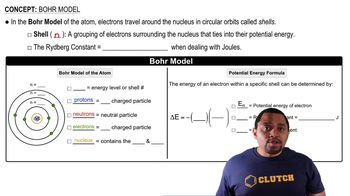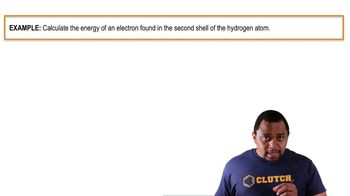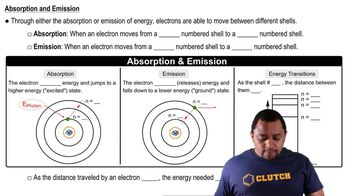Start typing, then use the up and down arrows to select an option from the list.
3:06 minutes
Problem 39
Textbook Question

# (a) Using Equation 6.5, calculate the energy of an electron in the hydrogen atom when n = 3 and when n = 6. Calculate the wavelength of the radiation released when an electron moves from n = 6 to n = 3. when n = 6Verified Solution
This video solution was recommended by our tutors as helpful for the problem above.
155views

### Watch next

Master Bohr Model with a bite sized video explanation from Jules Bruno

Start learning02:4301:2103:29